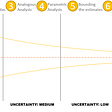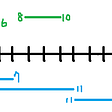# Asymptotic analysis of algorithms

Algorithm is a finite sequence of well-defined, computer-implementable instructions, typically to solve a class of problems or to perform a computation, and as anything else, we need a standard way to measure the performances of these algorithms to decide which ones to use in our programs.

In order to compare the performances of different algorithms, we need to look at several qualities of those algorithms: like the space it needs to use to apply that algorithm or how easy it is for the programmer to implement it. But more often than not, we tend to look at the running time of those algorithms, because let’s face it, nobody wants to wait.

While doing so, it is convenient to ignore the inputs of smaller sizes, because with the current technology we have today in our hands, there will be no problem at all , for example, to sort 500 integers. We are absolutely free to choose whatever the algorithm we want to implement, because it’ll not make that much of a difference. However, as the input size increases, performances of algorithms will play much more important role on how much one needs to wait to get the output one needs.

There are three types of analysis we can apply: we can look at the worst cases (sorting an inversely sorted array), average cases (that is how bench marks are done, it requires a little bit of knowledge of the domain you’re dealing with, so that an input similar to the average case can be analyzed) and best cases (which gives no clue whatsoever about the performance of the algorithm in a world where Murphy’s law holds)

As a result,

fast algorithms ≈ Slowly growing worst-case running time as input gets larger

Now, let’s look at the functions we can represent our running times with:

`╔═════════════════╦═════════════╗║     Function    ║ Explanation ║╠═════════════════╬═════════════╣║ f(n) = c        ║ Constant    ║║ f(n) = log(n)   ║ Logarithmic ║║ f(n) = n        ║ Linear      ║║ f(n) = n.log(n) ║ Superlinear ║║ f(n) = n^2      ║ Quadratic   ║║ f(n) = n^3      ║ Cubic       ║║ f(n) = c^n      ║ Exponential ║║ f(n) = n!       ║ Factorial   ║╚═════════════════╩═════════════╝`

Dominance relationship between these functions is as follows:

n! >> c^n>> n³ >> n² >> n.log(n) >> n >> log(n) >> 1

When analyzing algorithms, in order to avoid the details and have a general idea about the running time of an algorithm on a given input size, RAM (Random Access Machine) model is used. In this model, all arithmetic operations, all memory accesses and operations takes exactly one time step.

For example, if we look at the following example:

So the running time of the algorithm becomes:

But, even with a simple algorithm like this, running time function is somewhat complicated and hard to comprehend how it would behave as n grows for someone who looks at it. So instead of this, we try to find a function that bounds this function (above, below, or both) and get a more clear image.

These are the notations used for these functions that bounds the running time function for all values of input size n greater than or equal to a certain value.

So in order to simplify those complicated running time functions, we only keep the most dominant one (according to the relationship given above) and omit the rest, because as n gets larger, say log(n) for example will make a negligible contribution to the running time of the algorithm than n³, so it’ll not be that effective in identifying the performance of the algorithm.

If we look at the image above, I think we all can agree that the one we are most interested in would be big-oh function which represent an upper bound for the running time. For the image above for the second graph, we can say that there exists some positive constant c, when applied to function g(n), after a certain point(n₀), g(n) is always greater than f(n). In that case we say that

f(n) is order of g(n)

Example:

f(n) = 4n⁴ + 3 + 2 + 4n + 1 is O(n⁴).

Because 5n⁴+ 3n3 + 2n2 + n + 1 <= (4 + 3 + 2 + 1 + 1)n⁴ = c.n⁴ ,

for c = 15 and n₀ = 1.

We can also say f(n) is order-of n⁵ but we need to choose the smallest possible one as our big-oh, otherwise it may not be useful in comparing with other algorithms which is the whole point of why we’re doing this in the first place.

*Images are taken from Introduction to Algorithms Book, 3rd Edition by Cormen.,Leiserson, Rivest & Stein

--

--

--

## More from Mehmet Akcay

a geek who loves to understand the reasons behind things... and colors... Colors are cool.

Love podcasts or audiobooks? Learn on the go with our new app.

## R.O.M. Auctioneering; Accurately estimate complex projects in one hour.## Kafka Subscription — A Novice Way## So what’s about The Graph in all this future?## A complete guideline to Android mobile app development## A Quickie About Web Development For Beginners & The One’s Yet To Begin## Selecting GameObjects in Unity## Integrating Pub/Sub Lite with Apache Beam## Mehmet Akcay

a geek who loves to understand the reasons behind things... and colors... Colors are cool.

## Parallel, Distributed and Concurrent Systems## 56. Merge Intervals## Remove a node in Linked List without traversing it